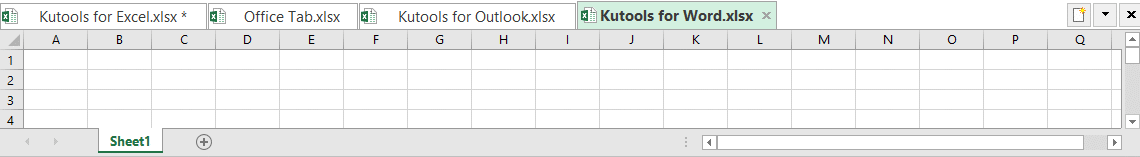## How to calculate cube root or Nth root in Excel?

In Excel, you can do many calculations, such as sum, average, count…, but, have you ever tried to calculate cube root or Nth root of some selected numbers in Excel as below screenshot shown? In this tutorial, I will introduce some easy tricks for you to deal with this task.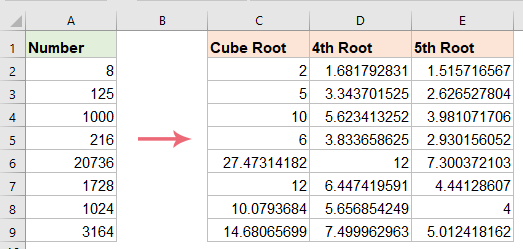#### Calculate cube root or Nth root with Exponential operator

Normally, the Exponential operator can help you to calculate the cube root or Nth root of numbers in a worksheet, please do as this:

1. Copy or enter the below formula into a blank cell:

=A2^(1/3)

2. And then drag the fill handle down to fill this formula to other cells, and the cube root of the specified cells are calculated as below screenshot shown: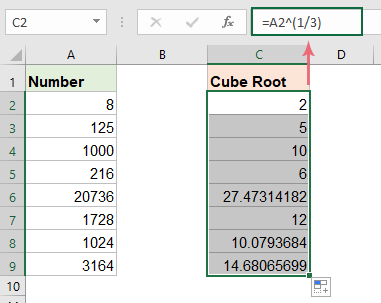Tips: To get the Nth root of the numbers, you just need to raise the number to the power 1/n. For instance, to get the 4th root, change n to 4, to get the 5th root, change n to 5, and so on…, =A2^(1/4), see screenshot: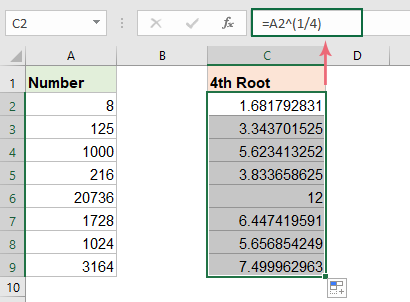#### Calculate cube root or Nth root with Power function

In Excel, you can also use the Power function to calculate the cube root or Nth root.

1. Copy or enter the following formula into a blank cell:

=POWER(A2,1/3)

2. Then drag the fill handle down to fill this formula to other cells to get the cube root results, see screenshot: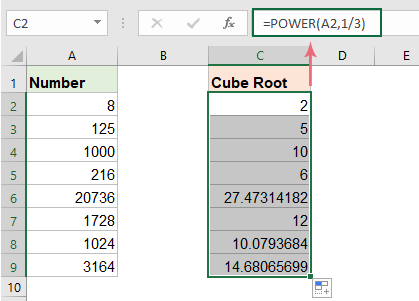Tips: To get the 4th, 5th or nth root of the numbers, please change the number 3 to 4, 5, n as you need.

#### Calculate cube root or Nth root with VBA code

Besides the above formulas, you can also create a VBA code to calculate the cube root or Nth root of numbers in Excel. To do this, please follow the steps below:

1. Hold down ALT + F11 keys to open the Microsoft Visual Basic for Applications window.

2. Click Insert > Module, and paste the following code in the Module Window.

VBA code: Calculate cube root of numbers

``````Sub cube_root()
'Updateby ExtendOffice
Dim xrng As Range
Dim xcell As Range
Dim x As Integer
Set xrng = Application.Range("A2:A9")
x = 1
For Each xcell In xrng
xcell.offset(0, x).Value = xcell ^ (1 / 3)
Next
End Sub
``````
Note: In the above code, A2:A9 is the list of cells that you want to calculate, the number 3 within the script xcell.offset(0, x).Value = xcell ^ (1 / 3) indicates the cube root to get, if you want to get the nth root, you just need to change it to n as you need.

3. Then, press F5 key to run this code, and the cube root results are calculated next to the number column as below screenshot shown: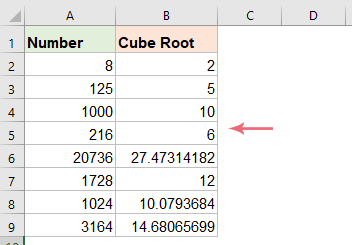## The Best Office Productivity Tools

### Kutools for Excel Solves Most of Your Problems, and Increases Your Productivity by 80%

• Super Formula Bar (easily edit multiple lines of text and formula); Reading Layout (easily read and edit large numbers of cells); Paste to Filtered Range...
• Merge Cells/Rows/Columns and Keeping Data; Split Cells Content; Combine Duplicate Rows and Sum/Average... Prevent Duplicate Cells; Compare Ranges...
• Select Duplicate or Unique Rows; Select Blank Rows (all cells are empty); Super Find and Fuzzy Find in Many Workbooks; Random Select...
• Exact Copy Multiple Cells without changing formula reference; Auto Create References to Multiple Sheets; Insert Bullets, Check Boxes and more...
• Favorite and Quickly Insert Formulas, Ranges, Charts and Pictures; Encrypt Cells with password; Create Mailing List and send emails...
• Extract Text, Add Text, Remove by Position, Remove Space; Create and Print Paging Subtotals; Convert Between Cells Content and Comments...
• Super Filter (save and apply filter schemes to other sheets); Advanced Sort by month/week/day, frequency and more; Special Filter by bold, italic...
• Combine Workbooks and WorkSheets; Merge Tables based on key columns; Split Data into Multiple Sheets; Batch Convert xls, xlsx and PDF...
• Pivot Table Grouping by week number, day of week and more... Show Unlocked, Locked Cells by different colors; Highlight Cells That Have Formula/Name...### Office Tab - brings tabbed interface to Office, and make your work much easier

• Enable tabbed editing and reading in Word, Excel, PowerPoint, Publisher, Access, Visio and Project.
• Open and create multiple documents in new tabs of the same window, rather than in new windows.
• Increases your productivity by 50%, and reduces hundreds of mouse clicks for you every day!